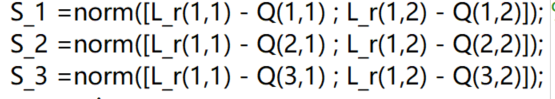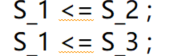# How to solve the error : sqrt( {convex}

,the S_1, S_2 and S_3 represent the distance respectively.

Use `norm`.

`S_1 = norm([L_r(1,1)-Q(1,1);L_r(1,2)-Q(1,2)])`

If you want to get fancier, you can use `norms` with a matrix argument, and calculate all the S_i 's at once, placed into a vector.

help cvx/norm

Disciplined convex programming information:
norm is convex, except when P<1, so an error will result if
these non-convex “norms” are used within CVX expressions. norm
is nonmonotonic, so its input must be affine.

help norms

norms Computation of multiple vector norms.
norms( X ) provides a means to compute the norms of multiple vectors
packed into a matrix or N-D array. This is useful for performing
max-of-norms or sum-of-norms calculations.

``````All of the vector norms, including the false "-inf" norm, supported
by NORM() have been implemented in the norms() command.
norms(X,P)           = sum(abs(X).^P).^(1/P)
norms(X)             = norms(X,2).
norms(X,inf)         = max(abs(X)).
norms(X,-inf)        = min(abs(X)).
If X is a vector, these computations are completely identical to
their NORM equivalents. If X is a matrix, a row vector is returned
of the norms of each column of X. If X is an N-D matrix, the norms
are computed along the first non-singleton dimension.

norms( X, [], DIM ) or norms( X, 2, DIM ) computes Euclidean norms
along the dimension DIM. norms( X, P, DIM ) computes its norms
along the dimension DIM.

Disciplined convex programming information:
norms is convex, except when P<1, so an error will result if these
non-convex "norms" are used within CVX expressions. norms is
nonmonotonic, so its input must be affine.``````

I want to express the distance S_1<S_2, and S_1<3, but the problem of {convex} <= {convex} arises.Those are non-convex constraints.

How to convert this non-convex constraint into a convex constraint

If those are the constraints you need, then you can try YALMIP (you will need to use `sqrtm` instead of `norm` for the non-convex usage).